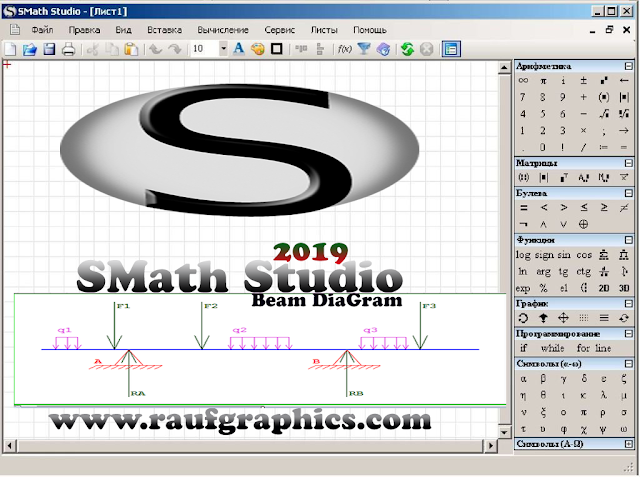## medianet# SMath Studio ®(Free) Mathematical Software:-

SMath Studio has many features for studying and solving the most complicated mathematical equations,with a simple layout and extensive features this is a great tool for nearly any level of math.
It has numerous computing options and awful user interface with an integrated mathematical reference book.
SMath Studio can be easily extended based on your needs. The built-in extensions manager tool allows to get access to hundreds official and third-party resources of the following types: handbooks and tutorials, handbooks and tutorials,usage examples, plug-ins, SMath Viewer based applications, interface translations, interactive books.
SMath Studio ® is a Mathematical program with page like GUI. It has an ability to work with systems matrices vectors complex numbers infinities and fractions equations. It allows creating standalone EXE files with all SMath Studio features enabled. Feature includes support for background images for peparsheets.

SMath Studio ® was designed to be a free version of PTC Mathcad. It can solve Differential Eq,Graphing Functions in two or three Dimensional symbolic calculations including solving systems of equations Matrix operations including determinants find roots of polynomials and functions symbolic and numeric differentiation of functions numeric integration simple multiline looped programs user defined functions and units of Measurement.

SMath Studio 0.98.6080.MSI
File Size 2. MB